# Vector Subtraction (Geometric): Formula & Examples

An error occurred trying to load this video.

Try refreshing the page, or contact customer support.

Coming up next: Standard Basis Vectors: Definition & Examples

### You're on a roll. Keep up the good work!

Replay
Your next lesson will play in 10 seconds
• 0:05 Why We Need to…
• 1:22 The Geometric Approach
• 3:08 Example
• 4:22 Lesson Summary

Want to watch this again later?

Timeline
Autoplay
Autoplay
Speed

#### Recommended Lessons and Courses for You

Lesson Transcript
Instructor: David Wood

David has taught Honors Physics, AP Physics, IB Physics and general science courses. He has a Masters in Education, and a Bachelors in Physics.

After watching this lesson, you will be able to subtract vectors geometrically and give examples of how that process might be used in physics. A short quiz will follow.

## Why We Need to Subtract Vectors

Subtracting two numbers that are scalars (that don't have a direction) is pretty easy: 10 apples - 6 apples = 4 apples. Really, that's all there is to it. But, when you add direction when you start working with vectors, things get a lot trickier. Yes, if you and your friend have a tug-of-war and he pulls with 10 newtons and you pull with 6 newtons, the result is an overall force of 4 newtons, just like with the apples. But, what if you're pulling at different angles?

Let's imagine that instead of a tug-of-war you're pushing a table at an angle of 40 degrees, and your friend is pulling at an angle of 75 degrees, each with different forces. Where does the table go? Suddenly, the sheer amount that you're pulling isn't all that's important. Now the direction matters, so we have to start adding and subtracting vectors.

Though very often adding vectors is more useful, subtracting can also be important. You just have to look for a physics equation that has a minus sign between two vector quantities to know when you might have to subtract. Here's an example: acceleration equals final velocity minus initial velocity divided by time. Time isn't a vector (that's just a number), but the final and initial velocities are both vectors. So, if we want to figure out the acceleration of an object, it will involve subtracting one vector from another.

## The Geometric Approach

There are two main ways of subtracting vectors: mathematically and geometrically. In this lesson, we're going to go through the geometric approach: subtracting vectors by positioning them end-to-end.

To avoid lots of messy math, the geometric approach has a nice simplicity to it - all you have to do is draw a diagram. Of course, you do have to draw a very accurate diagram: a scale drawing. A scale drawing is a drawing where the lengths and angles of every line relate to each other in a consistent way that matches the reality. So, for example, with a force vector, you could say that every centimeter equals a force of 10 newtons. So, a 50-newton force vector would be an arrow with a length of 5 centimeters.

To add two vectors that are drawn to scale (as described in another lesson) you position them tip to tail. To subtract two vectors you do something similar, but the one you're subtracting goes in the opposite direction. The orientation and length of the vector stays the same, but the direction reverses.

A vector v might look like this: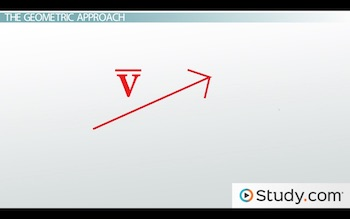But a vector negative v would look like this: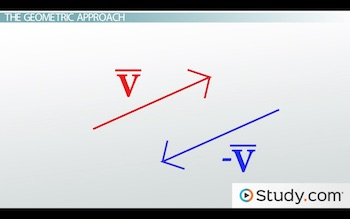Here, for example, we have two velocity vectors: the final velocity of a car and its initial velocity.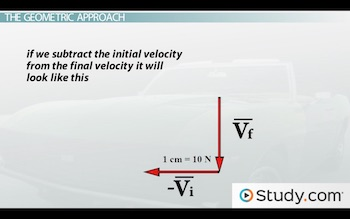If we subtract them like this, then to get the final vector, we draw a vector that goes from the very start to the very finish.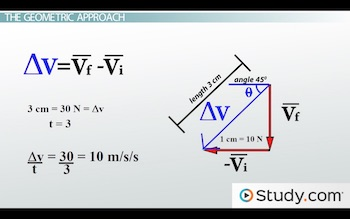Our final result, which we'll call delta v, is equal to the vector vf minus the vector vi.

We could then measure the length of our arrow in the scale drawing and the angle in which the arrow is pointing to figure out some numbers for our value of delta v. Last of all, we could divide the magnitude of our vector by t to get the acceleration since acceleration is vf minus vi divided by t.

## Example

Let's go through another example:

Imagine that you're trying to yank a cable out from behind a heavy desk. In the process of pulling on the cable to get it free you start pulling one way, and 3 seconds later you're pulling another way. You start pulling this way with a force of 3 newtons: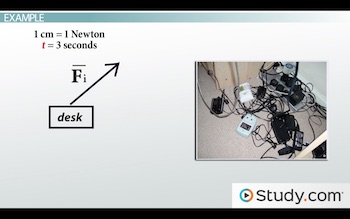And end up pulling this way with a force of 5 newtons by the time the 3 seconds are up: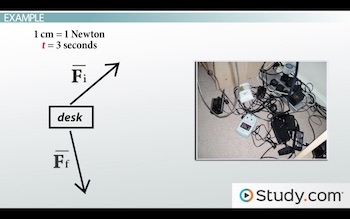To unlock this lesson you must be a Study.com Member.

### Register to view this lesson

Are you a student or a teacher?

### Unlock Your Education

#### See for yourself why 30 million people use Study.com

##### Become a Study.com member and start learning now.
Back
What teachers are saying about Study.com

### Earning College Credit

Did you know… We have over 160 college courses that prepare you to earn credit by exam that is accepted by over 1,500 colleges and universities. You can test out of the first two years of college and save thousands off your degree. Anyone can earn credit-by-exam regardless of age or education level.